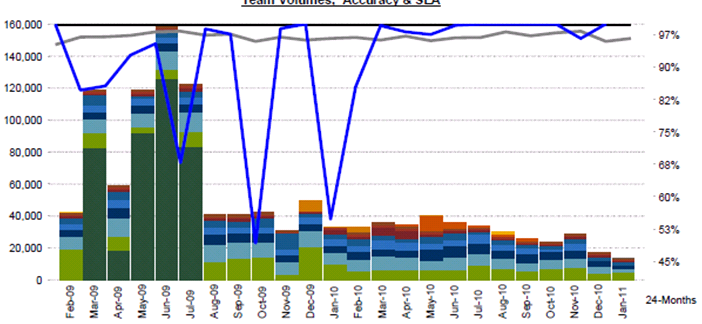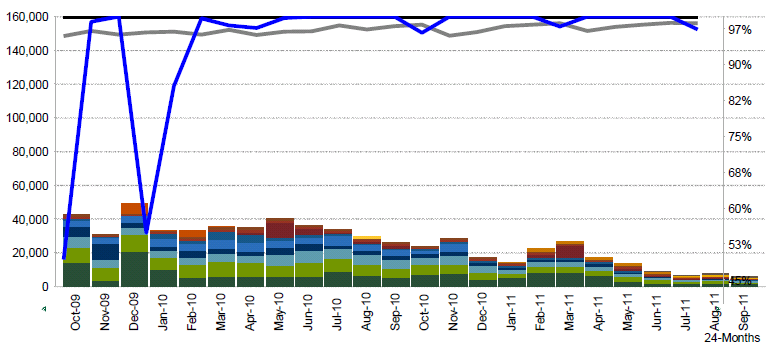# QlikView App Dev

Discussion Board for collaboration related to QlikView App Development.

Announcements
On May 18th at 10AM EDT we will answer your QlikView questions live. REGISTER
cancel
Showing results for
Did you mean:Not applicable

## Graph Problem

Hello,

Here's my graph last January 2011and here's my graph for this month. The scale is too high.Here's the expressions I used

Static Max:

=ceil(max(aggr(sum([Volumes]),[MMM-YY])),   pow(10,len(textbetween(round(max(aggr(sum([Volumes]),[MMM-YY]))),'','',1))-2))

Static Step:

=ceil(max(aggr(sum([Volumes]),[MMM-YY])),   pow(10,len(textbetween(round(max(aggr(sum([Volumes]),[MMM-YY]))),'','',1))-2))/8

Thanks,

Salie

5 RepliesMVP

Salie

Have you checked to see what these subexpressions evaluate to?

max(aggr(sum([Volumes]),[MMM-YY]))

and

pow(10,len(textbetween(round(max(aggr(sum([Volumes]),[MMM-YY]))),'','',1))-2))

If you need to see what values are returned by the aggregation:

concat(aggr(sum([Volumes]),[MMM-YY]), ',')

Regards

Jonathan

Logic will get you from a to b. Imagination will take you everywhere. - A EinsteinNot applicable
Author

Hi Jonathan,

concat(aggr(sum([Volumes]),[MMM-YY]), ',')?

Thanks,

SalieNot applicable
Author

Hi Jonathan,

concat(aggr(sum([Volumes]),[MMM-YY]), ',')?

Thanks,

SalieMVP

Salie

Create a text box, and enter the expression in the text box. Put an = in front of the expression.

Regards

Jonathan

Logic will get you from a to b. Imagination will take you everywhere. - A EinsteinNot applicable
Author

Hi,

I changed my expression to your expression. It worked. The only problem I have is that I need to show '50,000' after '45,000' to make it look complete.

I place the =concat expression you have provided on both Static Step and Static Max.thanks a lot!